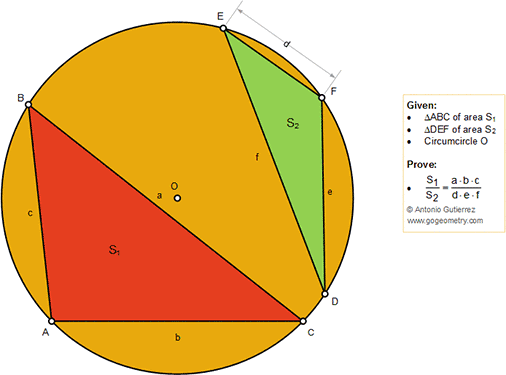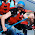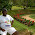## Friday, October 5, 2018

### Geometry Problem 1391: Two Triangles, Ratio of Areas, Circumcircle

Geometry Problem. Post your solution in the comment box below.
Level: Mathematics Education, High School, Honors Geometry, College.

Details: Click on the figure below.#### 2 comments:

1.According to the Law of Sines (https://en.wikipedia.org/wiki/Law_of_sines) a/sinA = 2R, so, S1 = 1/2*b*c*sinA = 1/2*a*b*c/2R = (a*b*c)/(4*R). Similarly S2 = (d*e*f)/(4*R) and S1/S2 = (a*b*c)/(d*e*f), Q.E.D.

2.Let h be the height of the perpendicular from A to BC.

If R is the circumradius, from similar triangles,
(c/2)/R = h/b and S1 = ha/2
Eliminating h, S1 = abc/4R
Similarly S2 = def/4R

Dividing, S1/S2 = abc/def

Sumith Peiris
Moratuwa
Sri Lanka